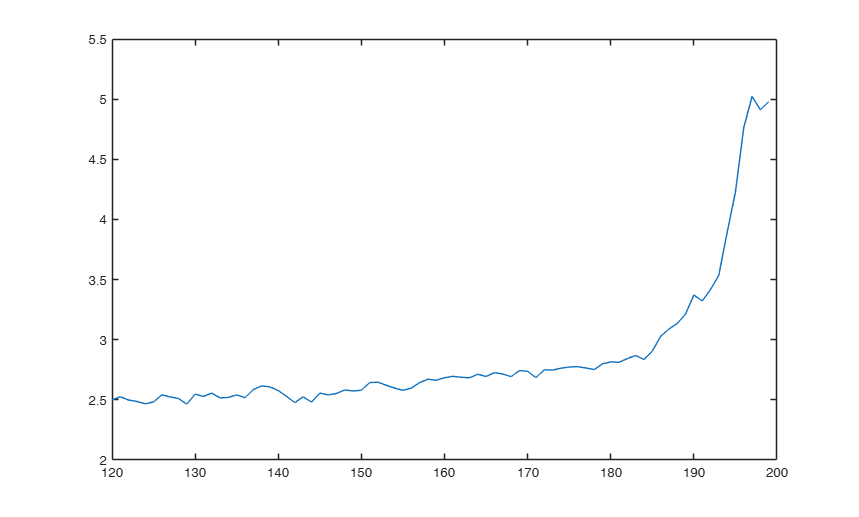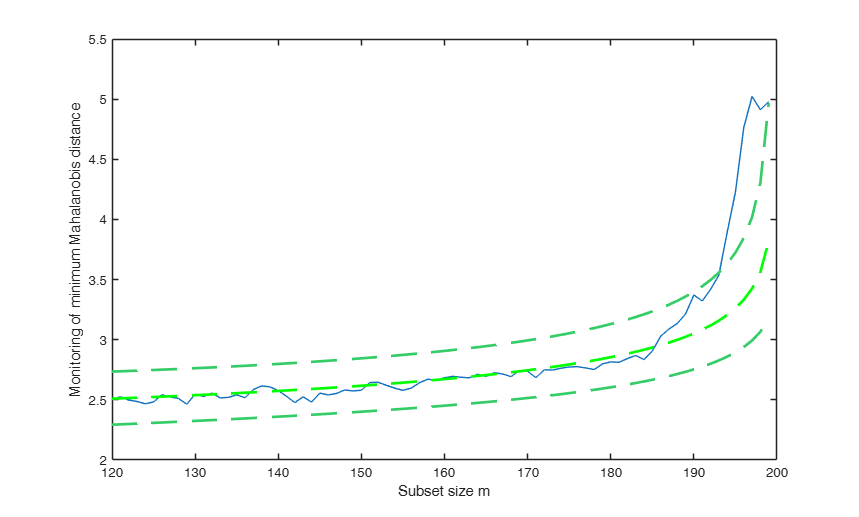# FSMmmd

FSMmmd monitors minMD

## Syntax

• mmd=FSMmmd(Y,bsb)example
• mmd=FSMmmd(Y,bsb,Name,Value)example
• [mmd,Un]=FSMmmd(___)example
• [mmd,Un,varargout]=FSMmmd(___)example

## Description

 mmd =FSMmmd(Y, bsb) Minimum Mahalanobis distance.

 mmd =FSMmmd(Y, bsb, Name, Value) FSMmmd with optional arguments.

 [mmd, Un] =FSMmmd(___) Checking the unit(s) included in the subset at each step of the search.

 [mmd, Un, varargout] =FSMmmd(___) Checking the units belonging to subset in each step of the search.

## Examples

expand all

###Minimum Mahalanobis distance.

Personalized initial subset (small n). Create an initial subset with the 4 observations which fell the smallest number of times outside the robust bivariate ellipses and with the lowest Mahalanobis Distance.

rng('default')
n=200;
v=3;
m0=4;
randn('state',123456);
Y=randn(n,v);
%Contaminated data
Ycont=Y;
Ycont(1:5,:)=Ycont(1:5,:)+3;
[fre]=unibiv(Y);
fre=sortrows(fre,[3 4]);
bs=fre(1:m0,1);
mmd=FSMmmd(Ycont,bs);
plot(mmd(:,1),mmd(:,2))###FSMmmd with optional arguments.

Plotting the bandwith of the minimum Mahalanobis distance

n=200;
v=3;
m0=4;
randn('state',123456);
Y=randn(n,v);
%Contaminated data
Ycont=Y;
Ycont(1:5,:)=Ycont(1:5,:)+3;
[fre]=unibiv(Y);
fre=sortrows(fre,[3 4]);
bs=fre(1:m0,1);
[mmd]=FSMmmd(Ycont,bs,'plots',1);
% The output is exactly the same as that of FSMmmdeasy
% mmd1=FSMmmdeasy(Ycont,bs,'plots',1);### Checking the unit(s) included in the subset at each step of the search.

% Un contains the unit(s) present in the new subset but not in the old one.
n=200;
v=3;
m0=4;
randn('state',123456);
Y=randn(n,v);
%Contaminated data
Ycont=Y;
Ycont(1:5,:)=Ycont(1:5,:)+3;
[fre]=unibiv(Y);
fre=sortrows(fre,[3 4]);
bs=fre(1:m0,1);
[mmd,Un]=FSMmmd(Ycont,bs,'plots',1);
% The output is exactly the same as that of FSMmmdeasy
% [mmd1,Un]=FSMmmdeasy(Ycont,bs,'plots',1);

### Checking the units belonging to subset in each step of the search.

Personalized initial subset (large n). Each row of BB matrix is associated to a unit while each colum is associated to a step of the fwd search.

n=20000;
v=3;
m0=10;
randn('state',123456);
Y=randn(n,v);
% 25 per cent of Contaminated data
Ycont=Y;
Ycont(1:5000,:)=Ycont(1:5000,:)+3;
[fre]=unibiv(Y);
fre=sortrows(fre,[3 4]);
bs=fre(1:m0,1);
[mmd,Un,BB]=FSMmmd(Ycont,bs,'plots',1);

## Input Arguments

### Y — Input data. Matrix.

n x v data matrix; n observations and v variables. Rows of Y represent observations, and columns represent variables.

Missing values (NaN's) and infinite values (Inf's) are allowed, since observations (rows) with missing or infinite values will automatically be excluded from the computations.

Data Types: single|double

### bsb — Units forming subset. Vector.

List of units forming the initial subset.

If bsb=0 (default) then the procedure starts with p units randomly chosen else if bsb is not 0 the search will start with m0=length(bsb)

Data Types: single | double

### Name-Value Pair Arguments

Specify optional comma-separated pairs of Name,Value arguments. Name is the argument name and Value is the corresponding value. Name must appear inside single quotes (' '). You can specify several name and value pair arguments in any order as  Name1,Value1,...,NameN,ValueN.

Example:  'init',50 , 'msg',0 , 'nocheck',false , 'plots',0 

### bsbsteps —Save the units forming subsets.vector.

It specifies for which steps of the fwd search it is necessary to save the units forming subsets. If bsbsteps is 0 we store the units forming subset in all steps. The default is store the units forming subset in all steps if n<=5000, else to store the units forming subset at steps init and steps which are multiple of 100. For example, as default, if n=7530 and init=6, units forming subset are stored for m=init, 100, 200, ..., 7500.

Example:  'bsbsteps',[100 200] stores the unis forming  subset in steps 100 and 200.

Data Types: double

### init —Point where to start monitoring required diagnostics.scalar.

Note that if bsb is supplied, init>=length(bsb). If init is not specified it will be set equal to floor(n*0.6).

Example:  'init',50 

Data Types: double

### msg —It controls whether to display or not messages about great interchange on the screen.scalar.

If msg==1 (default) messages are displyed on the screen else no message is displayed on the screen.

Example:  'msg',0 

Data Types: double

### nocheck —It controls wether to perform checks on matrix Y.boolean.

If nocheck is equal to true no check is performed on matrix Y. As default nocheck=false.

Example:  'nocheck',false 

Data Types: logical

### plots —It specify whether it is necessary to produce the plots of minimum Mahalanobis distance.scalar.

If plots=1, a plot of the monitoring of minMD among the units not belonging to the subset is produced on the screen with 1% 50% and 99% confidence bands else (default) no plot is produced.

Example:  'plots',0 

Data Types: double

## Output Arguments

### mmd —Monitoring of minimum Mahalanobis distance each step of the forward search. (n-init) -by- 2 matrix

1st col = fwd search index (from init to n-1);

2nd col = minimum Mahalanobis distance.

### Un —Unit(s) included in the subset at each step of the search. (n-init) -by- 11 Matrix

REMARK: in every step the new subset is compared with the old subset. Un contains the unit(s) present in the new subset but not in the old one.

Un(1,2) for example contains the unit included in step init+1.

Un(end,2) contains the units included in the final step of the search.

### varargout —Units belonging to subset in each step of the search. BB : n -by- (n-init+1) matrix

Each row is associated to a unit while each colum is associated to a step of the fwd search.

Atkinson, A.C., Riani, M. and Cerioli, A. (2004), "Exploring multivariate data with the forward search", Springer Verlag, New York.